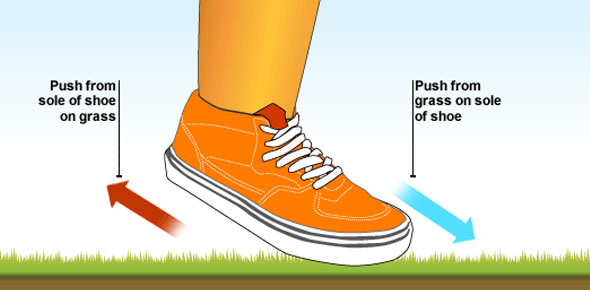# Describing And Measuring Motion Quiz (Motion)

13 Questions | Attempts: 1973SettingsMotion is a change in position of an object over time. After our classes this week you should be able to measure motion and know how it comes to be and how to measure a distance. Take the quiz below and test your understanding on describing and measuring motion. All the best!

• 1.
A reference point is a place or object used for comparison to determine if something is in motion.
• A.

True

• B.

False

• 2.
How many centimeters are in one meter?
• A.

10

• B.

100

• C.

1,000

• D.

10,000

• 3.
What would be the best unit to measure a flag pole?
• A.

Millimeter

• B.

Centimeter

• C.

Meter

• D.

Mile

• 4.
What measurements did we use for determining the speed of the Shake-N-Go cars?
• A.

Mi/min

• B.

Ft/sec

• C.

In/sec

• D.

Cm/sec

• 5.
Which of the following is the formula for calculating speed?
• A.

Time divided by distance

• B.

Distance minus time

• C.

Distance divided by time

• 6.
What would be the best unit to use to measure the tip of your pencil?
• A.

Feet

• B.

Kilometer

• C.

Millimeter

• D.

Meter

• 7.
Why is the International System of Units used by scientists around the world?
• A.

To communicate more clearly.

• B.

It is based on the number 10.

• C.

Calclations with this system are easy.

• D.

All of the above.

• 8.
What is the difference between speed and velocity?
• A.

There is no difference.

• B.

Speed has no direction but velocity does.

• C.

Speed is the measurement of direction.

• D.

None of the above.

• 9.
A weather forecaster may give the speed and velocity of a storm.
• A.

True

• B.

False

• 10.
What is the speed of something that moves 400 meters in 4 minutes?
• A.

10 m/min

• B.

100 m/min

• C.

10 mph

• D.

100 mph

• 11.
What is the speed of something that moves 3,600 cm in 40 sec?
• A.

90 cm/sec

• B.

900 cm/sec

• C.

9 cm/sec

• D.

None of the above

• 12.
What is the average of these 3 speeds: 78 mph, 59 mph, and 65 mph?
• A.

82.7

• B.

74.5

• C.

67.3

• D.

44.2

• 13.
What shoud you come to class prepared with everyday?
• A.

Planner, textbook, notebook

• B.

Pen or Pencil

• C.

Willingness to learn & Good manners

• D.

All of the above

## Related Topics

×

Wait!
Here's an interesting quiz for you.